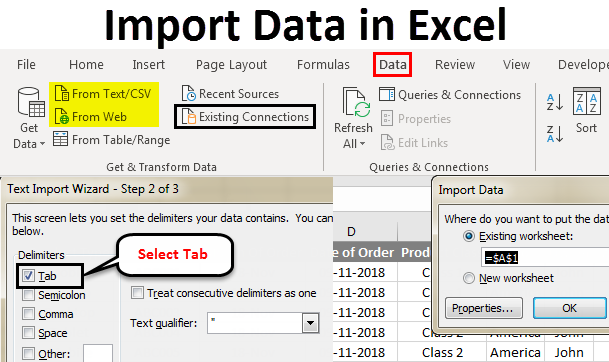# How to import data In Excel VBA

Oct 21, 2020 • edited Oct 23, 2020Below we will look at a program in Excel VBA that imports sheets from other Excel files into one Excel file.

Situation:Add the following code lines to the command button:

1. First, we declare two variables of type String, a Worksheet object and one variable of type Integer.

``````          Dim directory As String, fileName As String, sheet As Worksheet, total As Integer
``````

2. Turn off screen updating and displaying alerts.

``````          Application.ScreenUpdating = False

``````

3. Initialize the variable directory. We use the Dir function to find the first *.xl?? file stored in this directory.

``````          directory = &quot;c:\\test\\&quot;

fileName = Dir(directory &amp; &quot;*.xl??&quot;)
``````

Note: The Dir function supports the use of multiple character (*) and single character (?) wildcards to search for all different type of Excel files.

4. The variable fileName now holds the name of the first Excel file found in the directory. Add a Do While Loop.

``````          Do While fileName &lt;&gt; &quot;&quot;
``````

Loop

Add the following code lines (at 5, 6, 7 and 8) to the loop.

5. There is no simple way to copy worksheets from closed Excel files. Therefore we open the Excel file.

``````          Workbooks.Open (directory &amp; fileName)
``````

6. Import the sheets from the Excel file into import-sheet.xlsm.

``````          For Each sheet In Workbooks(fileName).Worksheets
``````

total = Workbooks("import-sheets.xlsm").Worksheets.count

Workbooks(fileName).Worksheets(sheet.Name).Copy _

after:=Workbooks("import-sheets.xlsm").Worksheets(total)

Next sheet

Explanation: the variable total holds track of the total number of worksheets of import-sheet.xlsm. We use the Copy method of the Worksheet object to copy each worksheet and paste it after the last worksheet of import-sheets.xlsm.

7. Close the Excel file.

``````          Workbooks(fileName).Close
``````

8. The Dir function is a special function. To get the other Excel files, you can use the Dir function again with no arguments.

``````          fileName = Dir()
``````

Note: When no more file names match, the Dir function returns a zero-length string (""). As a result, Excel VBA will leave the Do While loop.

9. Turn on screen updating and displaying alerts again (outside the loop).

``````          Application.ScreenUpdating = True